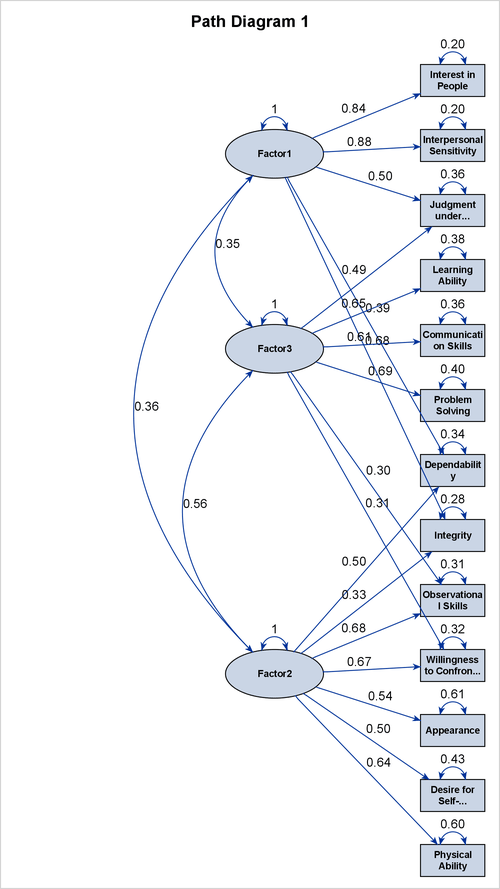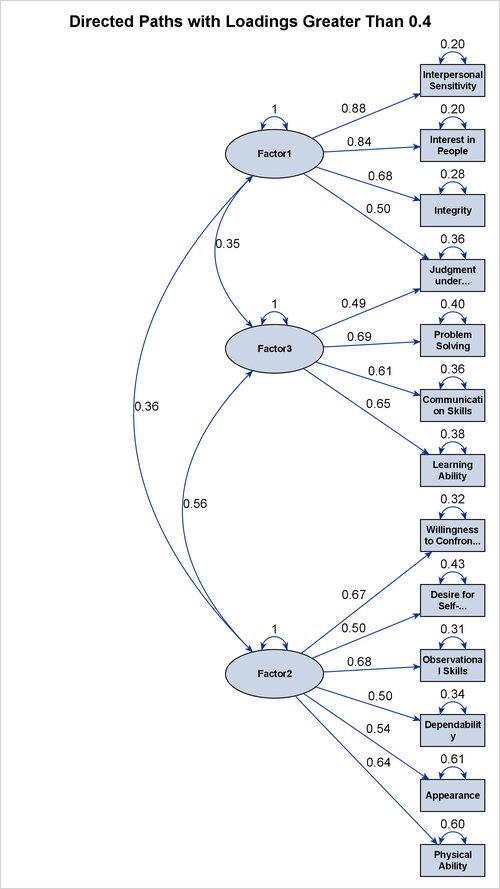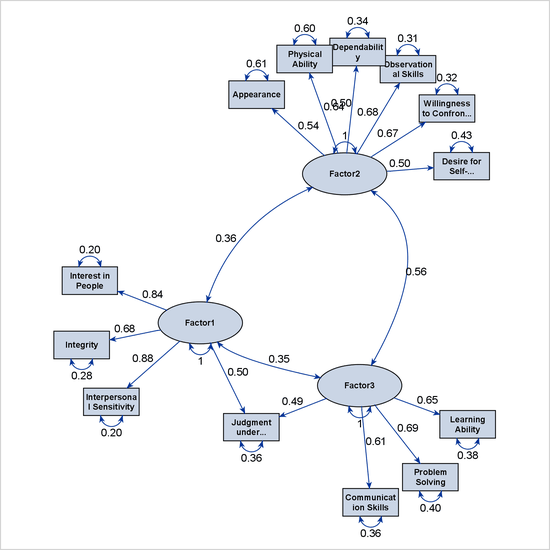# The FACTOR Procedure

### Example 37.5 Creating Path Diagrams for Factor Solutions

The section Getting Started: FACTOR Procedure analyzes a data set that contains 14 ratings of 103 police officers to demonstrate some basic techniques in factor analysis. To illustrate the creation and uses of path diagrams, this example analyzes this data set again by using the following statements:

```ods graphics on;

proc factor data=jobratings(drop='Overall Rating'n)
priors=smc rotate=quartimin plots=pathdiagram;
run;
```

The PRIORS=SMC option specifies that the squared multiple correlations are to be used as the prior communality estimates. As a result, the factors are extracted by the principal factor method. The ROTATE=QUARTIMIN option requests the use of the quartimin rotation to obtain the final factor solution. The PLOTS=PATHDIAGRAM option requests a path diagram for the final solution.

Except for the PLOTS=PATHDIAGRAM option, previous examples have already described the FACTOR options that are used in this example. Therefore, this example focuses only on the creation of path diagrams.

Output 37.5.1 and Output 37.5.2 show the quartimin-rotated factor correlations and factor pattern, respectively.

Output 37.5.1: Quartimin-Rotated Factor Correlations

Inter-Factor Correlations
Factor1 Factor2 Factor3
Factor1 1.00000 0.36103 0.34823
Factor2 0.36103 1.00000 0.55721
Factor3 0.34823 0.55721 1.00000

Output 37.5.2: Quartimin-Rotated Factor Pattern

Rotated Factor Pattern (Standardized Regression Coefficients)
Factor1 Factor2 Factor3
Communication Skills 0.21280 0.13541 0.61091
Problem Solving 0.17222 0.01424 0.68767
Learning Ability -0.09904 0.24961 0.65430
Judgment under Pressure 0.49876 -0.02005 0.48879
Observational Skills -0.15748 0.67661 0.30273
Willingness to Confront Problems -0.19106 0.66639 0.31135
Interest in People 0.84249 0.12734 -0.00710
Interpersonal Sensitivity 0.87832 -0.12964 0.15116
Desire for Self-Improvement 0.19078 0.49891 0.23297
Appearance 0.05254 0.53846 0.10857
Dependability 0.39241 0.50035 0.12032
Physical Ability 0.14404 0.63901 -0.15220
Integrity 0.68277 0.32719 -0.01887

Output 37.5.3 shows the path diagram for the quartimin-rotated factor solution. The path diagram represents correlations among factors by double-headed links or paths. For example, Output 37.5.3 represents the correlation between `Factor1` and `Factor2` by a curved doubled-headed link. The numerical value, 0.36, is the correlation between the two factors, as can be verified from the table in Output 37.5.1. Similarly, Output 37.5.3 shows other factor correlations by curved doubled-headed links.

Output 37.5.3: Default Path Diagram for the Quartimin-Rotated SolutionThe path diagram in Output 37.5.3 also represents factor variances and error variances by double-headed links. However, each of these links points to an individual variable, rather than to a pair of variables as the double-headed links for correlations do. The path diagram also displays the numerical values of factor variances or error variances next to the associated links.

The directed links from factors to variables in the path diagram represent the effects of factors on the variables. The path diagram displays the numerical values of these effects, which are the loading estimates that are shown in Output 37.5.2. However, to aid the interpretation of the factors, the path diagram does not show all factor loadings or their corresponding links. By default, the path diagram displays only the links that have loadings greater than 0.3 in magnitude. For example, instead of associating `Factor1` with all variables, the path diagram in Output 37.5.3 displays only five directed links from `Factor1` to the variables. The weaker links that have loadings less than 0.3 are not shown.

The use of the 0.3 loading value (or greater in magnitude) for relating factors to variables is referred to as the "0.3-rule" in the field of factor analysis. However, this is only a convention, and sometimes you might want to use a different criterion to interpret the factors. For example, the path diagram in Output 37.5.3 shows that variables `Dependability`, `Integrity`, and `Observational Skills` are all associated with more than one factor. Hence, factors might not be interpreted unambiguously.

One way to tackle this interpretation problem is to set a stricter criterion for interpreting factors. You can use the FUZZ= option to set such a criterion. For example, you specify the following PATHDIAGRAM statement to display only the strong directed links that are associated with a 0.4 or greater magnitude in the loading estimates:

```pathdiagram fuzz=0.4 title='Directed Paths with Loadings Greater Than 0.4';
```

The preceding statement also uses the TITLE= option to specify a customized title for the path diagram. Output 37.5.4 shows the resulting path diagram. In this path diagram, only one observed variable is linked to two factors. All other observed variables link to unique factors. Therefore, compared to the path diagram in Output 37.5.3, the path diagram in Output 37.5.4 provides a much "cleaner" picture for interpreting the factors.

Output 37.5.4: Path Diagram Showing Strong LinksThe current example has 13 observed variables in the path diagram. By default, PROC FACTOR uses the process-flow algorithm to lay out the variables. However, when the number of observed variables becomes large, the process-flow algorithm needs a lot of vertical space to align all observed variables in a vertical line. Displaying such a "long" path diagram in limited space (for example, in a page) might compromise the clarity of the path diagram.

To handle this issue, PROC FACTOR switches to the GRIP algorithm when the number of variables is greater than 14. However, you can override the layout algorithm whenever you find it useful to do so. For example, the ARRANGE=GRIP option in the following PATHDIAGRAM statement requests that the GRIP algorithm be used:

```pathdiagram fuzz=.4 arrange=grip notitle;
```

The NOTITLE option suppresses the display of the title for the path diagram. Output 37.5.5 shows the resulting path diagram, which spreads out the variables instead of aligning them vertically, as it does when it uses the process-flow algorithm in Output 37.5.4.

Output 37.5.5: Path Diagram Showing Strong Links by Using the ARRANGE=GRIP AlgorithmFor more information about the options for customizing path diagrams, see the PATHDIAGRAM statement.